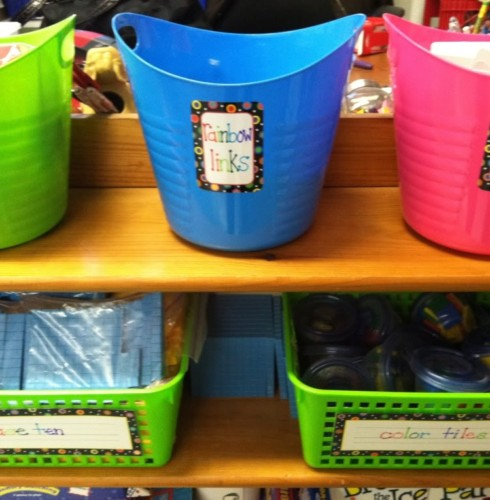## Author Allison TredwayIn 3rd grade, students are expected to generate simple equivalent fractions. Example: 1/2 = 4/8 and 4/6 = 2/3. Models should be used to help students identify equivalent fractions. Sentence strips or strips of paper cut into congruent rectangles can…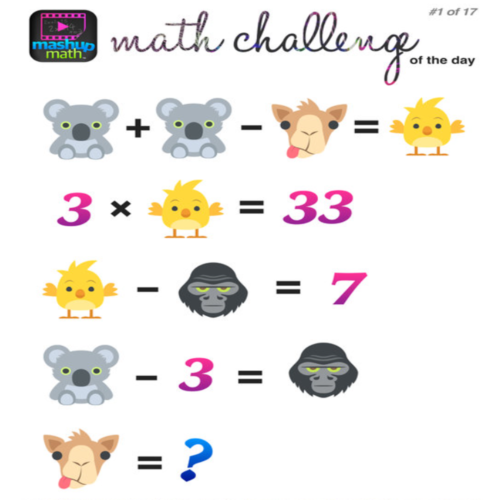Check out the website MashUp Math for awesome math challenges! These are great way to get students excited about math and stretch their algebraic thinking!In 3rd grade, students are expected to compare two fractions that have either the same numerator or the same denominator. When comparing fractions, it is important for students to understand the value of the fraction and consider both the numerator…What is multiplication? Multiplication is when we know the number of groups and the size of the equal groups, but the total amount is unknown. Students are expected to use the actions in a story problem to directly model what…What is fact fluency? An important goal for third graders is to develop fluency with multiplication and division by the end of the school year. Fact fluency is the ability to think of numbers flexibly and respond accurately in about…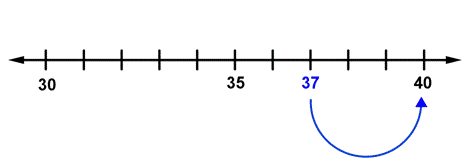Five or more raise the score, four or less give it a rest! This saying and others similar to it are often used to help students remember how to round numbers. When these students are asked why they rounded 48…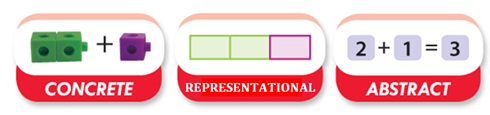What is the Concrete-Representational-Abstract (CRA) instructional approach? CRA is a gradual systematic approach to teaching mathematics. Each stage builds on to the previous stage and therefore must be taught in sequence. This three step approach has been found to be…What is direct modeling? Direct modeling is when students show exactly what is happening in the problem, using manipulatives or pictures, to solve. Direct modeling is a powerful strategy that provides a foundation for the more advanced strategies that follow.…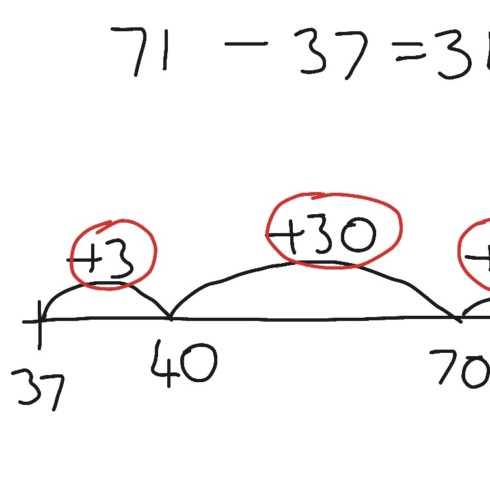What are open number lines? An open number line starts as a line with no numbers. They can be used as a visual representation for recording thinking during the process of mental computation. Why are open number lines so useful?…